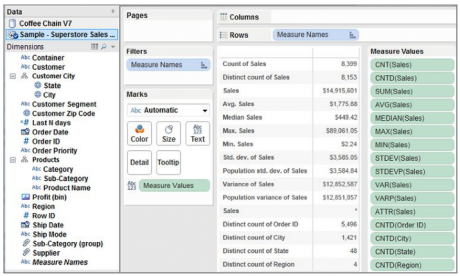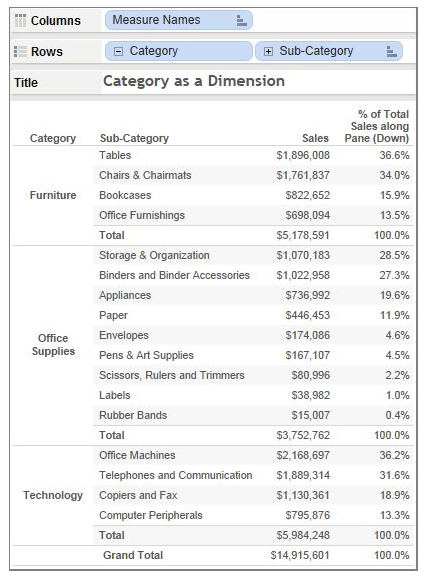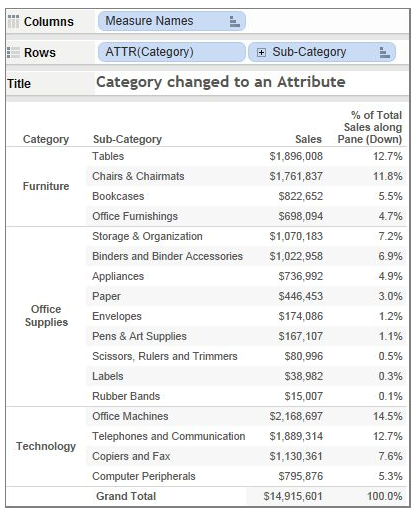Home / Tableau

# Tableau Aggregate Functions

Rating: 5.0Views: 38999
by Saikumar

Sometimes it is useful to look at numerical data in an aggregated form such as a summation or an average. The mathematical functions that produce aggregated data are called aggregation functions. Aggregation functions perform a calculation on a set of values and return a single value.

You can use Tableau to set an aggregation only for measures in relational data sources. Multidimensional data sources contain aggregated data only.

Aggregation defines how values are expressed. Most Tableau functions are calculated at the database server with only the results being sent to Tableau. If you are familiar with SQL, you will find most of the functions in Tableau are an extension of SQL. Tableau uses the Sum aggregation by default. If the default aggregation isn’t what you want, point at the pill of the measure you’ve placed into the view—right-click, and select more appropriate aggregation functions.

 Want to Learn Advanced Calculations & Chart Techniques to Create Innovative Analysis & Dashboards? Then enroll in Mindmajixs' MindMajix Tableau e learning

Here are the types of Tableau Aggregate Functions:

## Tableau Functions List

• Sum – Returns the sum of the numbers in a measure. Null values are ignored.
• Average – Returns the arithmetic mean of the numbers in a measure. Null values are ignored.
• Median – Returns the median of the numbers in a measure. Null values are ignored.
• Count – Returns the number of rows in a measure or a dimension. When applied to a dimension, Tableau creates a new temporary column that is a measure because the result of a count is a number. You can count numbers, dates, Booleans, and strings. Null values are ignored in all cases
• Count Distinct – Returns the number of unique values in a measure or dimension. When applied to a dimension, Tableau creates a new temporary column that is a measure because the result of a count is a number. You can count numbers, dates, Booleans, and strings. Null values are ignored in all cases.
• Minimum – Returns the smallest number in a measure or continuous dimension. Null values are ignored.
• Maximum – Returns the largest number in a measure or a continuous dimension. Null values are ignored.
• Standard Deviation – Returns the standard deviation of all values in the given expression based on a sample population. Null values are ignored. Returns a Null if there are fewer than 2 members in the sample that are not Null. Use this function if your data represents a sample of the population.
• Standard Deviation of a Population – Returns the standard deviation of all values in the given expression based on a biased population. Assumes that its arguments consist of the entire population. Use this function for large sample sizes.
• Variance – Returns the variance of all values in the given expression based on a sample. Null values are ignored. Returns a Null if there are fewer than 2 members in the sample that are not Null. Use this function if your data represent a sample of the population.
• The variance of a Population – Returns the variance of all values in the given expression based on a biased population. Assumes that its arguments consist of the entire population. Use this function for large sample sizes.

These are clearly defined in Tableau’s online manual. Search the help menu to read more about each of them if you are unfamiliar with the type of aggregation each provides.

 Related Article: Tableau Basics### Count Distinct Versus Count

One of the aggregate functions you can use in Tableau is Count (Distinct), which returns the number of unique values in a field.

These functions count records in different ways. Consider a data set that includes 10,000 records with 20 different regions. Performing a Count Distinct on the Region field returns a value of 20. The purpose of Count Distinct is to count the unique instances of a particular item. A Count aggregation of 10,000 records will result in an answer of 10,000 only because it counts all records.

Count Distinct is supported by relational database sources but is not supported by Excel, Access, or text files. You can add the ability to create Count Distinct aggregation when accessing those sources by performing a data extract. Tableau’s extract files do support Count Distinct aggregation.

### Median

Most data sources and all extracts support MEDIAN in Tableau as a standard aggregation.

However, Median is not supported by a direct connection from Tableau to Excel, Access, or text files. Performing a data extract will once again give you the ability to compute median values. Using the Superstore data set, figure 4.1 shows a crosstab displaying, all of the different aggregations available for the sales field in the data set.Different aggregation of sales Diagram

Notice that the bottom four rows are expressing Count Distinct values for different dimensions. By dragging each of those dimension fields into the crosstab using the right mouse button, the Count Distinct aggregation is expressed for each dimension. As you can see the data set includes over 5,000 different orders; over 1,400 cities; 48 states; and four regions.

### Dimension versus Attribute

When converted to an attribute, the dimension becomes just a label. In other words, it is not used to partition the data.

Aggregation behavior can be changed by altering the default method by which Tableau expresses dimensions. Figure 4.2 shows a crosstab containing sales by product category and sub-category. A table calculation is being used to display the percent of total sales that each row represents within each product category pane.

By default, Tableau partitions the result by the category dimension. Subtotals have been added by using the main menu option analysis/ totals, then showing subtotal and column totals. You can see that in each category pane the number of sales and percent of sales is totaled. But, if the category dimension is changed to an attribute, the category dimension will become a label only and no longer cause the data to be partitioned. Figure 4.3 shows the same dataset but with the category, the field changed to an attribute.

 Related Article: Tableau Interview Questions and Answers for ExperiencedDiagram of Product category as a dimensionDiagram of Product category as an attribute

The view still shows the light gray boundary lines between each category, but because the category dimension has been changed to an attribute, it will no longer partition the view. The sales total reflects the total for the entire crosstab and the percent of total sales is now expressing the percentage of total sales, but not the sales within each category. This may appear to be trivial, but as your skills advance and you begin to employ more advanced table calculations you get to understand how attributes change Tableau’s behavior.

List Of Tableau Courses: